Updating search results...

# 51 Results

View
Selected filters:
• CCSS.Math.Content.7.EE.B.4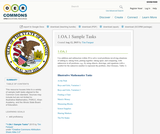Conditional Remix & Share Permitted
CC BY-SA
Rating
0.0 stars

This resource houses links to a variety of sample math tasks aligned to the Common Core standard. Sources may include but are not limited to: Illustrative Mathematics, PARCC, Khan Academy, and the Illinois State Board of Education.

Subject:
Mathematics
Material Type:
Assessment
Homework/Assignment
Lecture
Teaching/Learning Strategy
Author:
Tim Farquer
Date Added:
01/28/2016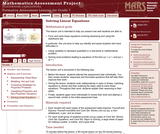Only Sharing Permitted
CC BY-NC-ND
Rating
5.0 stars

This lesson unit is intended to help teachers assess how well students are able to: form and solve linear equations involving factorizing and using the distributive law. In particular, this unit aims to help teachers identify and assist students who have difficulties in: using variables to represent quantities in a real-world or mathematical problem and solving word problems leading to equations of the form px + q = r and p(x + q) = r.

Subject:
Algebra
Material Type:
Assessment
Lesson Plan
Provider:
Shell Center for Mathematical Education
Provider Set:
Mathematics Assessment Project (MAP)
Date Added:
04/26/2013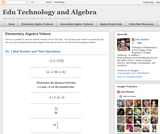Conditional Remix & Share Permitted
CC BY-NC
Rating
0.0 stars

Visually searchable database of Algebra 1 videos. Click on a problem and watch the solution on YouTube. Copy and paste this material into your CMS. Videos accompany the open Elementary Algebra textbook published by Flat World Knowledge.

Subject:
Algebra
Material Type:
Lecture
Lecture Notes
Provider:
Individual Authors
Provider Set:
Individual Authors
Author:
John Redden
Date Added:
04/29/2012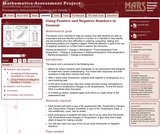Only Sharing Permitted
CC BY-NC-ND
Rating
3.5 stars

This lesson unit is intended to help teachers assess how well students are able to understand and use directed numbers in context. It is intended to help identify and aid students who have difficulties in ordering, comparing, adding, and subtracting positive and negative integers. Particular attention is paid to the use of negative numbers on number lines to explore the structures: starting temperature + change in temperature = final temperature final temperature Đ change in temperature = starting temperature final temperature Đ starting temperature = change in temperature.

Subject:
Numbers and Operations
Material Type:
Assessment
Lesson Plan
Provider:
Shell Center for Mathematical Education
Provider Set:
Mathematics Assessment Project (MAP)
Date Added:
04/26/2013Only Sharing Permitted
CC BY-NC-ND
Rating
4.5 stars

This lesson unit is intended to help assess how well students are able to interpret and use scale drawings to plan a garden layout. This involves using proportional reasoning and metric units.

Subject:
Algebra
Geometry
Ratios and Proportions
Material Type:
Assessment
Lesson Plan
Provider:
Shell Center for Mathematical Education
Provider Set:
Mathematics Assessment Project (MAP)
Date Added:
04/26/2013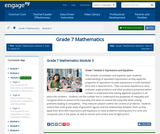Conditional Remix & Share Permitted
CC BY-NC-SA
Rating
0.0 stars

This module consolidates and expands upon students understanding of equivalent expressions as they apply the properties of operations to write expressions in both standard form and in factored form.  They use linear equations to solve unknown angle problems and other problems presented within context to understand that solving algebraic equations is all about the numbers.  Students use the number line to understand the properties of inequality and recognize when to preserve the inequality and when to reverse the inequality when solving problems leading to inequalities.  They interpret solutions within the context of problems.  Students extend their sixth-grade study of geometric figures and the relationships between them as they apply their work with expressions and equations to solve problems involving area of a circle and composite area in the plane, as well as volume and surface area of right prisms.

Subject:
Algebra
Material Type:
Module
Provider:
New York State Education Department
Provider Set:
EngageNY
Date Added:
11/15/2013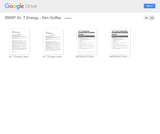Conditional Remix & Share Permitted
CC BY-NC
Rating
0.0 stars

Students will investigate different types of renewable and nonrenewable energy as they evaluating competing claims about humans' sources of power.

Subject:
Physical Science
Material Type:
Activity/Lab
Lesson Plan
Provider:
South Metro-Salem STEM Partnership
Author:
Ken Guffey
Date Added:
07/02/2015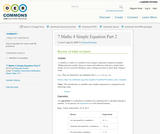Conditional Remix & Share Permitted
CC BY-SA
Rating
0.0 stars

Use of equation for some real life problems.

Subject:
Mathematics
Material Type:
Reading
Author:
Peeyush Kumar
Date Added:
01/28/2016Conditional Remix & Share Permitted
CC BY-NC
Rating
0.0 stars

Students first create a diagram that represents the distance a ship drops in each of a series of locks. Students create their diagrams based on a video of an actual ship traveling through the locks. Students need to use contextual clues in order to determine the relative drops in each of the locks.Key ConceptsStudents are expected to use the mathematical skills they have acquired in previous lessons or in previous math courses. The lessons in this unit focus on developing and refining problem-solving skills.Students will:Try a variety of strategies to approaching different types of problems.Devise a problem-solving plan and implement their plan systematically.Become aware that problems can be solved in more than one way.See the value of approaching problems in a systematic manner.Communicate their approaches with precision and articulate why their strategies and solutions are reasonable.Make connections between previous learning and real-world problems.Create efficacy and confidence in solving challenging problems in a real-world setting.Goals and Learning ObjectivesRead and interpret maps, graphs, and diagrams.Solve problems that involve linear measurement.Estimate length.Critique a diagram.

Subject:
Algebra
Material Type:
Lesson Plan
Provider:
Pearson
Date Added:
09/21/2015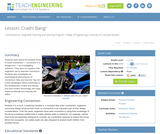Read the Fine Print
Educational Use
Rating
0.0 stars

Students learn about the physical force of linear momentum movement in a straight line by investigating collisions. They learn an equation that engineers use to describe momentum. Students also investigate the psychological phenomenon of momentum; they see how the "big mo" of the bandwagon effect contributes to the development of fads and manias, and how modern technology and mass media accelerate and intensify the effect.

Subject:
Engineering
Physics
Material Type:
Activity/Lab
Lesson Plan
Provider:
TeachEngineering
Provider Set:
TeachEngineering
Author:
Ben Heavner
Chris Yakacki
Denise Carlson
Malinda Schaefer Zarske
Date Added:
09/18/2014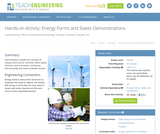Read the Fine Print
Educational Use
Rating
0.0 stars

Demonstrations explain the concepts of energy forms (sound, chemical, radiant [light], electrical, atomic [nuclear], mechanical, thermal [heat]) and states (potential, kinetic).

Subject:
Engineering
Physics
Material Type:
Activity/Lab
Provider:
TeachEngineering
Provider Set:
TeachEngineering
Author:
Jan DeWaters
Susan Powers
Date Added:
09/18/2014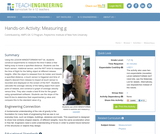Read the Fine Print
Educational Use
Rating
0.0 stars

Using the LEGO MINDSTORMS(TM) NXT kit, students construct experiments to measure the time it takes a free falling body to travel a specified distance. Students use the touch sensor, rotational sensor, and the NXT brick to measure the time of flight for the falling object at different release heights. After the object is released from its holder and travels a specified distance, a touch sensor is triggered and time of object's descent from release to impact at touch sensor is recorded and displayed on the screen of the NXT. Students calculate the average velocity of the falling object from each point of release, and construct a graph of average velocity versus time. They also create a best fit line for the graph using spreadsheet software. Students use the slope of the best fit line to determine their experimental g value and compare this to the standard value of g.

Subject:
Engineering
Physics
Material Type:
Activity/Lab
Provider:
TeachEngineering
Provider Set:
TeachEngineering
Author:
Jennifer Haghpanah
Keeshan Williams
Nicole Abaid
Date Added:
09/18/2014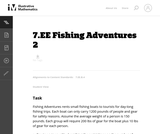Unrestricted Use
CC BY
Rating
0.0 stars

This task is the second in a series of three tasks that use inequalities in the same context at increasing complexity in 6th grade, 7th grade and in HS algebra. Students write and solve inequalities, and represent the solutions graphically.

Subject:
Mathematics
Algebra
Material Type:
Activity/Lab
Provider:
Illustrative Mathematics
Provider Set:
Illustrative Mathematics
Author:
Illustrative Mathematics
Date Added:
05/01/2012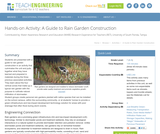Read the Fine Print
Educational Use
Rating
0.0 stars

Students are presented with a guide to rain garden construction in an activity that culminates the unit and pulls together what they have learned and prepared in materials during the three previous associated activities. They learn about the four vertical zones that make up a typical rain garden with the purpose to cultivate natural infiltration of stormwater. Student groups create personal rain gardens planted with native species that can be installed on the school campus, within the surrounding community, or at students' homes to provide a green infrastructure and low-impact development technology solution for areas with poor drainage that often flood during storm events.

Subject:
Engineering
Hydrology
Material Type:
Activity/Lab
Provider:
TeachEngineering
Provider Set:
TeachEngineering
Author:
Brigith Soto
Jennifer Butler
Krysta Porteus
Maya Trotz
Ryan Locicero
William Zeman
Date Added:
09/18/2014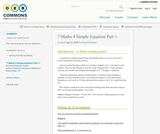Conditional Remix & Share Permitted
CC BY-SA
Rating
0.0 stars

This resource gives intro to equations

Subject:
Mathematics
Material Type:
Reading
Author:
Peeyush Kumar
Date Added:
01/28/2016Conditional Remix & Share Permitted
CC BY-NC
Rating
0.0 stars

Students discover how the addition and multiplication properties of inequality differ from the addition and multiplication properties of equality.Students use the addition and multiplication properties of inequality to solve inequalities. They graph their solutions on the number line.Key ConceptsIn this lesson, students extend their knowledge of inequalities from Grade 6. In Grade 6, students learned that solving an inequality meant finding which values made the inequality true. Students used substitution to determine whether a given value made an inequality true. They also used a number line to graph the solutions of inequalities. By graphing these solutions on a number line, they saw that an inequality has an infinite number of solutions.Now, in Grade 7, students work with inequalities that also contain negative numbers and learn to solve and graph solutions for inequalities such as −2x − 4 < 5. This involves first understanding how the properties of inequality differ from the properties of equality. When multiplying (or dividing) both sides of an inequality by the same negative number, the relationship between the two sides of the inequality changes, so it is necessary to reverse the direction of the inequality sign in order for the inequality to remain true. Once students understand this, they can apply the same steps they used to solve equations to solve inequalities, but remembering to reverse the direction of the inequality sign when multiplying or dividing both sides of the inequality by a negative number.Goals and Learning ObjectivesAccess prior knowledge of how to solve an inequality.Observe that when multiplying or dividing both sides of an inequality by the same negative number, the inequality sign must change direction.Solve and graph inequalities of the form px + q > r or px + q < r, where p, q, and r are specific rational numbers.

Subject:
Algebra
Material Type:
Lesson Plan
Provider:
Pearson
Date Added:
09/21/2015Conditional Remix & Share Permitted
CC BY-NC
Rating
0.0 stars

Students solve a problem about a salesperson's compensation. They solve the problem first by arithmetic and then by writing and solving an inequality.Key ConceptsIn Lesson 11, students learned how to solve inequalities using the addition and multiplication properties of inequality. In this lesson, they solve word problems by writing and solving inequalities.To help students make connections and see how problems can be solved in different ways, students first solve the same problem using arithmetic.Goals and Learning ObjectivesWrite and solve an algebraic inequality to solve a word problem.

Subject:
Algebra
Material Type:
Lesson Plan
Provider:
Pearson
Date Added:
09/21/2015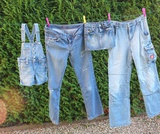Conditional Remix & Share Permitted
CC BY-NC
Rating
0.0 stars

Students use inequalities to solve real-world problems. They see that the solution of the algebraic inequality may differ from the solution to the problem it represents. For example, a fractional number or a negative number may not be an appropriate solution for a word problem.Students complete a Self Check. They are given an algebraic inequality that they need to solve. They then write and solve a word problem that the inequality could represent.Key ConceptsIn this lesson, students write and solve an algebraic inequality that matches a situation given in a word problem. They then interpret that algebraic solution in the context of the problem. For example, students write and solve an algebraic inequality to represent the number of T-shirts that can be bought given a certain amount of money and another purchase. The inequality produces the solution t < 2.5. Since a fractional part of a T-shirt does not make sense, students reason that 2 is the greatest number of T-shirts that can be purchased.Goals and Learning ObjectivesInterpret the solution to an algebraic inequality within the context of a word problem.

Subject:
Algebra
Material Type:
Lesson Plan
Provider:
Pearson
Date Added:
09/21/2015Conditional Remix & Share Permitted
CC BY-NC
Rating
0.0 stars

Students work in pairs to critique and improve their work on the Self Check. Students complete a task similar to the Self Check with a partner.Key ConceptsTo critique and improve the task from the Self Check and to complete a similar task with a partner, students use what they know about solving inequalities, graphing their solutions, and relating the inequalities to a real-world situation.Goals and Learning ObjectivesSolve algebraic inequalities.Graph the solutions of inequalities using number lines.Write word problems that match algebraic inequalities.Interpret the solution of an inequality in terms of a word problem.

Subject:
Algebra
Material Type:
Lesson Plan
Provider:
Pearson
Date Added:
09/21/2015Conditional Remix & Share Permitted
CC BY-NC
Rating
0.0 stars

Students explore the relationship between the flapping frequency, the amplitude, and the cruising speeds of a variety of animals to calculate their Strouhal numbers.Key ConceptsStudents are expected to use the mathematical skills they have acquired in previous lessons or in previous math courses. The lessons in this unit focus on developing and refining problem-solving skills. Students will:Try a variety of strategies to approaching different types of problems.Devise a problem-solving plan and implement their plan systematically.Become aware that problems can be solved in more than one way.See the value of approaching problems in a systematic manner.Communicate their approaches with precision and articulate why their strategies and solutions are reasonable.Make connections between previous learning and real-world problems.Create efficacy and confidence in solving challenging problems in a real-world setting.Goals and Learning ObjectivesAnalyze the relationship between the variables in an equation.Write formulas to show how variables relate.Communicate findings using multiple representations including tables, charts, graphs, and equations.

Subject:
Algebra
Material Type:
Lesson Plan
Provider:
Pearson
Date Added:
09/21/2015